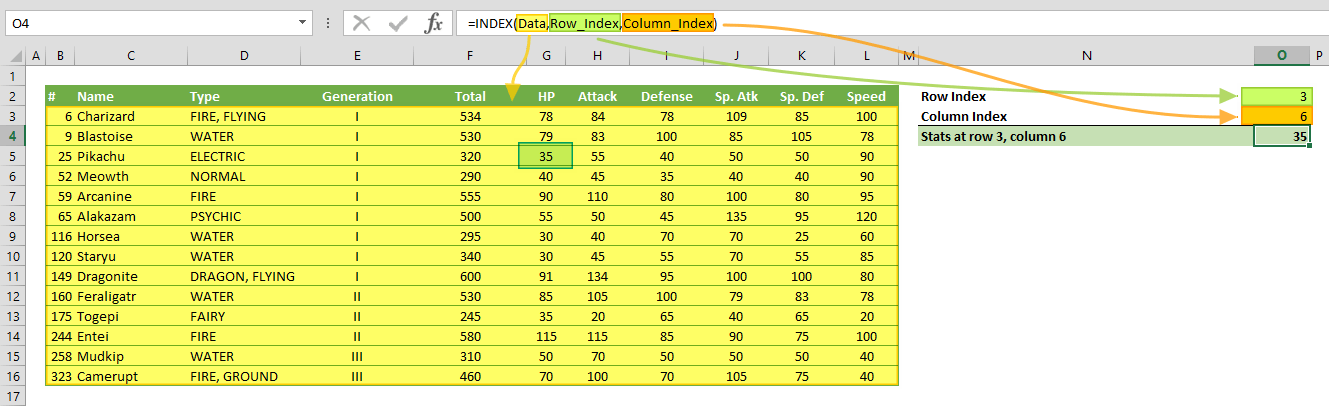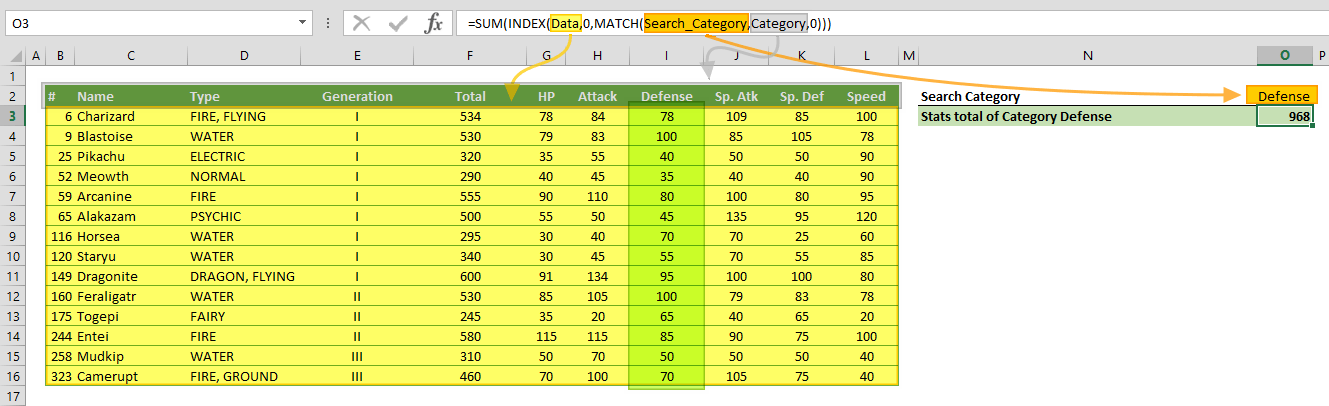The Excel INDEX function returns a value or the reference of a value at a given position in a range or array. The INDEX function has two different forms to return a value or a reference. Using an array form returns a value, while a reference form returns a reference. In this guide, we’re going to show you how to use the Excel INDEX function and also go over some tips and error handling methods.

# Supported versions

• All Excel Versions

# Excel INDEX Function Syntax

Array Form: INDEX(array, row_num, [column_num])

Reference Form: INDEX(reference, row_num, [column_num], [area_num])

# Arguments

 array / reference A range of cells or an array constant. row_num The position of the row in the reference or array. column_row Optional. The position of the column in the reference or array. area_num Optional. Specifies the area that is used in the formula.  Areas are numbered by the order they are specified. For example, if reference is (A1:C5,E10:G16) area_num 1 refers the range A1:C5 and area_num 2 refers the range E10:G16.

# Examples

Note that we're using named ranges in our examples to make the formulas easier to read. This is not required.

## Array Form

### Example 1

=INDEX(Data,Row_Index,Column_Index)
formula returns the value from the range Data where Row_Index and Column_Index intersect.### Example 2

=INDEX(Data,MATCH(Search_Name,Name,0),MATCH(Search_Category,Category,0))
formula is a common use case of collaboration INDEX and MATCH functions. The MATCH functions in row and column arguments determine the position of the values for the INDEX match.### Example 3

=SUM(INDEX(Data,0,MATCH(Search_Category,Category,0)))
formula is an example of returning array of numbers by the INDEX function. If you omit or enter 0 either row_num or col_num arguments, the INDEX function returns all values in omitted row or column.

In this example, the INDEX returns the values from the Defense column and the SUM function sums these numbers.## Reference Form

### Example 1

=INDEX((HP,Defense),Row_Index,1,Area_Index)
function uses area_num argument which is viable for the reference form of the INDEX function. In the example, the reference argument contains two ranges in the form of named ranges HP and Defense. Since the area_num argument is 2, the INDEX function evaluates the second area (range) in given references. As a result, it returns 40 which is the value of the third row of the named range Defense.### Example 2

=SUM(G3:INDEX(Data,MATCH(Search_Name,Name,0),MATCH(Search_Category,Category,0)))
formula uses the INDEX function in the reference structure. The reference in the SUM function starts from a single cell. However, the other side of the reference is a formula that is based on the same INDEX function. The INDEX function finds the cell reference whose coordinates are specified in the MATCH functions. As a result, the reference of the SUM function becomes, G3:I5 and the SUM function adds all the values in the reference.# Tips

• The Excel INDEX function can be combined with the MATCH. Combination of the two functions will give you a dynamic lookup approach: INDEX & MATCH: A Better Way to Look Up Data
• If the array argument contains only a single row or column, you can omit the corresponding row_num or column_num argument. For example,
=INDEX(A1:J1,3)
formula returns the value from the 3rd column.

# Issues

## #REF!

If values of row_num, column_num or area_num arguments are greater than the specified range, array, or the reference, the formula returns an #REF! error.

## #VALUE!

• If area_num is 0, the formula returns #VALUE!
• If values of row_num, column_num or area_num arguments are negative numbers, the formula returns #VALUE! error.